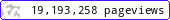•••••MadAsMaths.com :: I.Y.G.B. Practice Papers :: Undergraduate Methods Papers :: Mathematical Methods 2 Practice Papers

I.Y.G.B. MATHEMATICAL METHODS 2 PRACTICE PAPERS

These I.Y.G.B. practice papers follow the most common syllabi for a first year/second semester degree in Mathematics, Engineering or Science.

The examined topics are listed at the front cover of each paper.

All papers have model solutions.

 mathmeth2_a.pdfmathmeth2_a_solutions.pdfmathmeth2_b.pdfmathmeth2_b_solutions.pdfmathmeth2_c.pdfmathmeth2_c_solutions.pdfmathmeth2_d.pdfmathmeth2_d_solutions.pdfmathmeth2_e.pdfmathmeth2_e_solutions.pdfmathmeth2_f.pdfmathmeth2_f_solutions.pdfmathmeth2_g.pdfmathmeth2_g_solutions.pdfmathmeth2_h.pdfmathmeth2_h_solutions.pdfmathmeth2_i.pdfmathmeth2_i_solutions.pdfmathmeth2_j.pdfmathmeth2_j_solutions.pdfmathmeth2_k.pdfmathmeth2_k_solutions.pdfmathmeth2_l.pdfmathmeth2_l_solutions.pdfmathmeth2_m.pdfmathmeth2_m_solutions.pdfmathmeth2_n.pdfmathmeth2_n_solutions.pdfmathmeth2_o.pdfmathmeth2_o_solutions.pdfmathmeth2_p.pdfmathmeth2_p_solutions.pdfmathmeth2_q.pdfmathmeth2_q_solutions.pdfmathmeth2_r.pdfmathmeth2_r_solutions.pdfmathmeth2_s.pdfmathmeth2_s_solutions.pdfmathmeth2_t.pdfmathmeth2_t_solutions.pdfmathmeth2_u.pdfmathmeth2_u_solutions.pdfmathmeth2_v.pdfmathmeth2_v_solutions.pdfmathmeth2_w.pdfmathmeth2_w_solutions.pdfmathmeth2_x.pdfmathmeth2_x_solutions.pdfmathmeth2_y.pdfmathmeth2_y_solutions.pdfmathmeth2_z.pdfmathmeth2_z_solutions.pdf